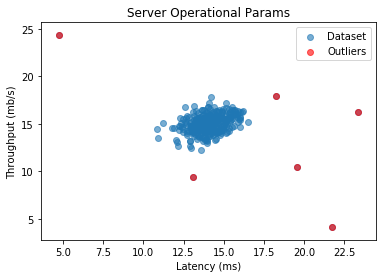# Anomaly Detection Using Gaussian Distribution¶

☝Before moving on with this demo you might want to take a look at:

Anomaly detection (also outlier detection) is the identification of rare items, events or observations which raise suspicions by differing significantly from the majority of the data.

The normal (or Gaussian) distribution is a very common continuous probability distribution. Normal distributions are important in statistics and are often used in the natural and social sciences to represent real-valued random variables whose distributions are not known. A random variable with a Gaussian distribution is said to be normally distributed and is called a normal deviate.

Demo Project: In this demo we will build a model that will find anomalies in server operational parameters such as Latency and Throughput.

In :
# To make debugging of logistic_regression module easier we enable imported modules autoreloading feature.
# By doing this you may change the code of logistic_regression library and all these changes will be available here.

import sys
sys.path.append('../..')


### Import Dependencies¶

• pandas - library that we will use for loading and displaying the data in a table
• numpy - library that we will use for linear algebra operations
• matplotlib - library that we will use for plotting the data
• anomaly_detection - custom implementation of anomaly detection using Gaussian distribution.
In :
# Import 3rd party dependencies.
import numpy as np
import pandas as pd
import matplotlib.pyplot as plt

# Import custom Gaussian anomaly detection implementation.


In this demo we will use the dataset with server operational parameters such as Latency and Throughput and will try to find anomalies in them.

In :
# Load the data.

# Print the data table.

Out:
Latency (ms) Throughput (mb/s) Anomaly
0 13.046815 14.741152 0
1 13.408520 13.763270 0
2 14.195915 15.853181 0
3 14.914701 16.174260 0
4 13.576700 14.042849 0
5 13.922403 13.406469 0
6 12.822132 14.223188 0
7 15.676366 15.891691 0
8 16.162875 16.202998 0
9 12.666451 14.899084 1
In :
# Print histograms for each feature to see how they vary.
histohrams = pd_data[['Latency (ms)', 'Throughput (mb/s)']].hist(grid=False, figsize=(10,4))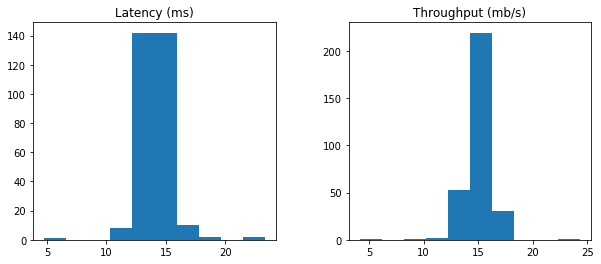### Plot the Data¶

Let's plot Throughput(Latency) dependency and see if the distribution is similar to Gaussian one.

In :
# Extract first two column from the dataset.
data = pd_data[['Latency (ms)', 'Throughput (mb/s)']].values

# Plot the data.
plt.scatter(data[:, 0], data[:, 1], alpha=0.6)
plt.xlabel('Latency (ms)')
plt.ylabel('Throughput (mb/s)')
plt.title('Server Operational Params')
plt.show()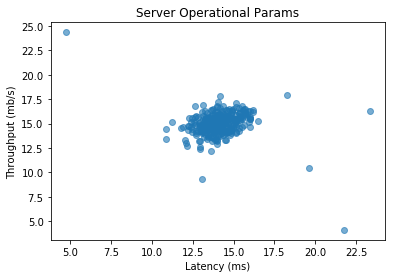### Find Anomalies Using Gaussian Distribution¶

Let's try to use our custom anomaly detection implementation using Gaussian distribution.

In :
# Init Gaussian anomaly instance.
gaussian_anomaly = GaussianAnomalyDetection(data)

# Let's see Gaussian estimation parameters.
print('mu')
print(gaussian_anomaly.mu_param)

print('\n')

print('sigma^2')
print(gaussian_anomaly.sigma_squared)

mu
[14.11222578 14.99771051]

sigma^2
[1.83263141 1.70974533]


### Visualize the Fit¶

Let's draw a contour plots that will represent our Gaussian distribution for the dataset.

In :
# Create a 3D grid to build a contour plots.

# Create ranges along X and Y axes.
latency_from = 0
latency_to = 35

throughput_from = 0
throughput_to = 35

step = 0.5

latency_range = np.arange(latency_from, latency_to, step)
throughput_range = np.arange(throughput_from, throughput_to, step)

# Create X and Y grids.
(latency_grid, throughput_grid) = np.meshgrid(latency_range, throughput_range)

# Flatten latency and throughput grids.
flat_latency_grid = latency_grid.flatten().reshape((latency_grid.size, 1))
flat_throughput_grid = throughput_grid.flatten().reshape((throughput_grid.size, 1))

# Joing latency and throughput flatten grids together to form all combinations of latency and throughput.
combinations = np.hstack((flat_latency_grid, flat_throughput_grid))

# Now let's calculate the probabilities for every combination of latency and throughput.
flat_probabilities = gaussian_anomaly.multivariate_gaussian(combinations)

# Resghape probabilities back to matrix in order to build contours.
probabilities = flat_probabilities.reshape(latency_grid.shape)

# Let's build plot our original dataset.
plt.scatter(data[:, 0], data[:, 1], alpha=0.6)
plt.xlabel('Latency (ms)')
plt.ylabel('Throughput (mb/s)')
plt.title('Server Operational Params')

# On top of our original dataset let's plot probability contours.
plt.contour(latency_grid, throughput_grid, probabilities, levels=10)

# Display the plot.
plt.show()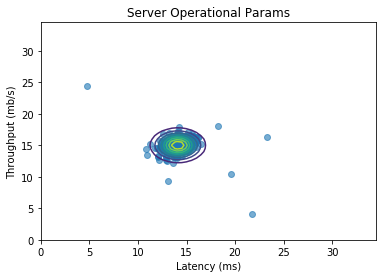### Select best threshold¶

Now, in order to decide which examples should be counted as an anomaly we need to decide which probability threshold to choose. We could do it intuitively but since we have all data examples labeled in our dataset let's use that data to calculate the best threshold.

In :
# Extract the information about which example is anomaly and which is not.
num_examples = data.shape
labels = pd_data['Anomaly'].values.reshape((num_examples, 1))

# Returns the density of the multivariate normal at each data point (row) of X dataset.
probabilities = gaussian_anomaly.multivariate_gaussian(data)

# Let's go through many possible thresholds and pick the one with the highest F1 score.
(epsilon, f1, precision_history, recall_history, f1_history) = gaussian_anomaly.select_threshold(
labels, probabilities
)

print('Best epsilon:')
print(epsilon)

print('\n')

print('Best F1 score:')
print(f1)

Best epsilon:
8.986095083415364e-05

Best F1 score:
0.8


### Plot Precision/Recall Progress¶

Let's now plot precision, reacall and F1 score changes for every iteration.

In :
# Make the plot a little bit bigger than default one.
plt.figure(figsize=(15, 5))

# Plot precission history.
plt.subplot(1, 3, 1)
plt.xlabel('Iteration')
plt.ylabel('Value')
plt.title('Precission Progress')
plt.plot(precision_history)

# Plot recall history.
plt.subplot(1, 3, 2)
plt.xlabel('Iteration')
plt.ylabel('Value')
plt.title('Recall Progress')
plt.plot(recall_history)

# Plot F1 history.
plt.subplot(1, 3, 3)
plt.xlabel('Iteration')
plt.ylabel('Value')
plt.title('F1 Progress')
plt.plot(f1_history)

# Display all plots.
plt.show()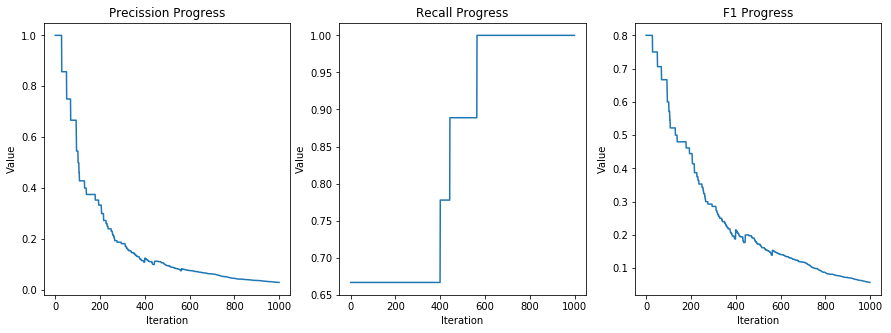### Fing Outliers¶

Since now we have calculated best epsilon we may find outliers.

In :
# Find indices of data examples with probabilities less than the best epsilon.
outliers_indices = np.where(probabilities < epsilon)

# Plot original data.
plt.scatter(data[:, 0], data[:, 1], alpha=0.6, label='Dataset')
plt.xlabel('Latency (ms)')
plt.ylabel('Throughput (mb/s)')
plt.title('Server Operational Params')

# Plot the outliers.
plt.scatter(data[outliers_indices, 0], data[outliers_indices, 1], alpha=0.6, c='red', label='Outliers')

# Display plots.
plt.legend()
plt.plot()

Out:
[]